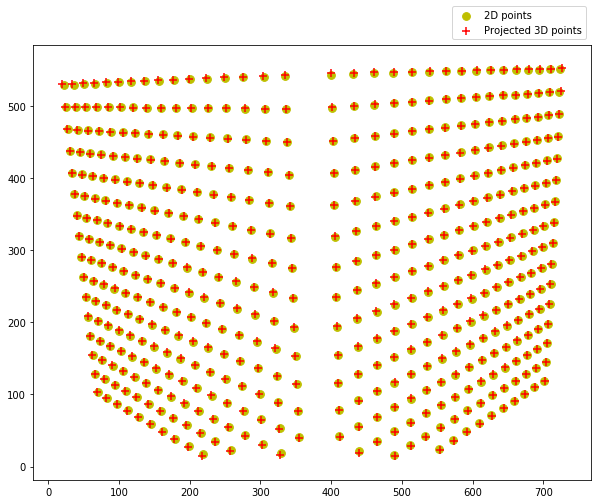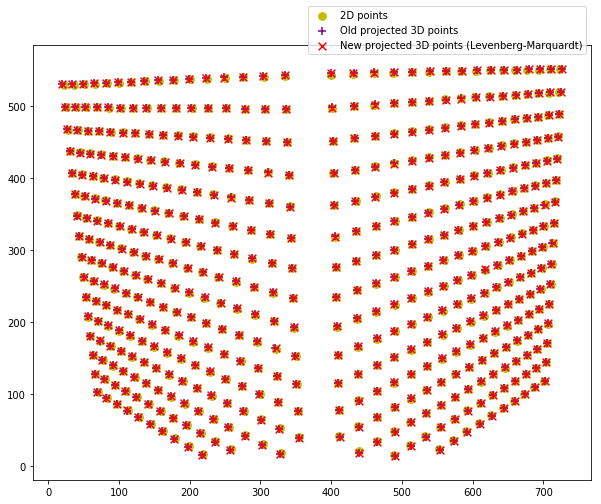# TP Calibration¶

To execute a notebook, you will need to install jupyter. If you are using anaconda (strongly advised). If you cannot/don't want to use notebooks, you can return both your python code and a report in pdf. Note that all the TPs will use python2. If you are using python3, you might need to do a few changes to the provided code.

## 0. Imports¶

In :
import numpy as np
# this is the key library for manipulating arrays. Use the online ressources! http://www.numpy.org/

import matplotlib.pyplot as plt
# used to read images, display and plot http://matplotlib.org/api/pyplot_api.html .
#You can also check this simple intro to using ipython notebook with images https://matplotlib.org/users/image_tutorial.html

%matplotlib inline
# to display directly in the notebook

import scipy.ndimage as ndimage
# one of several python libraries for image procession

import scipy.io as sio

import scipy.optimize as optimize
# usefull for optimization

plt.rcParams['image.cmap'] = 'gray'
# by default, the grayscale images are displayed with the jet colormap: use grayscale instead


In :
data=sio.loadmat('clean_points.mat')
pts2d=data['pts2d']
pts3d=data['pts3d']


1: Build the matrix that define the calibration equation

In :
def calibr_mat(pts2d=pts2d, pts3d=pts3d):
A = np.zeros((2*pts3d.shape, 12))

for i in range(pts3d.shape):
A[2*i, 4:7] = -pts3d[i, :]
A[2*i, 7] = -1
A[2*i, 8:11] = pts2d[i, 1]*pts3d[i,:]
A[2*i, 11] = pts2d[i, 1]

A[2*i+1,:3] = pts3d[i, :]
A[2*i+1, 3] = 1
A[2*i+1, 8:11] = -pts2d[i, 0]*pts3d[i,:]
A[2*i+1, 11] = -pts2d[i, 0]

return A

A = calibr_mat()


2: Perform SVD (using np.linalg.svd). Check that the SVD is coherent and that one of the singular value is close to 0. Compute the projection matrix.

In :
U, s, V = np.linalg.svd(A)

S = np.zeros((U.shape, V.shape))
S[:12, :12] = np.diag(s)
print(np.isclose(np.dot(U, np.dot(S, V)), A))

P = V[-1,:].reshape(3, 4)

[[ True  True  True ...,  True  True  True]
[ True  True  True ...,  True  True  True]
[ True  True  True ...,  True  True  True]
...,
[ True  True  True ...,  True  True  True]
[ True  True  True ...,  True  True  True]
[ True  True  True ...,  True  True  True]]


3: Compute the error between the projected 3D points and the 2D points.

In :
N = pts3d.shape
proj_pts3d = np.hstack((pts3d, np.ones(N).reshape(N, 1)))
proj_pts2d = np.hstack((pts2d, np.ones(N).reshape(N, 1)))

sum((pts2d[i, 0] - np.dot(P, proj_pts3d[i, :]/np.dot(P, proj_pts3d[i, :])))**2 \
+ (pts2d[i, 1] - np.dot(P, proj_pts3d[i, :])/np.dot(P, proj_pts3d[i, :]))**2 for i in range(N))

Out:
450.01475379292839

4: Visualize the projected 3D points and the 2D points on the same figure (use plt.scatter)

In :
proj_pts = P.dot(proj_pts3d.T).T

fig = plt.figure(figsize=(10,8))

plt.scatter(*zip(*pts2d), label='2D points', s=60, c='y')
plt.scatter(*zip(*[(p/p, p/p) for p in proj_pts]),\
s=60, label='Projected 3D points', marker='+', c='red')
plt.legend(bbox_to_anchor=(1, 1.1))
plt.show()5: Extract the intrinsic and extrinsic parameters of the camera from the matrix (use np.linalg.inv)

$$P ≝ \left[M \;\middle|\; b\right] = K \left[R \;\middle|\; t\right]$$

where

• $$K ≝ \begin{pmatrix}α & γ & u_0 \\ 0 & β & v_0 \\ 0 & 0 & 1\end{pmatrix}$$
• $$M = KR ⟹ R = K^{-1}M$$
• $$Kt = b ⟹ t = K^{-1}b$$

$$MM^T = KK^T = \begin{pmatrix}α^2 + γ^2 + u_0^2 & γβ+u_0 v_0 & u_0 \\ γβ+u_0 v_0 & β^2 + v_0^2 & v_0 \\ u_0 & v_0 & 1\end{pmatrix}$$

In :
# Intrinsic parameters: alpha, beta, gamma, u_0, v_0

MM_T = np.linalg.inv(P[:, :-1]).dot(np.linalg.inv(P[:, :-1]).T)
# normalizing
MM_T /= MM_T[2,2]

u_0, v_0 = MM_T[0,2], MM_T[1,2]
beta = np.sqrt(MM_T[1,1]-v_0**2)
gamma = (MM_T[0,1]-u_0*v_0)/beta
alpha = np.sqrt(MM_T[0,0]-u_0**2-gamma**2)
K = np.array([[alpha, gamma, u_0], [0, beta, v_0], [0, 0, 1]])

# Extrinsic parameters: R, t

K_inv = np.linalg.inv(K)
R = K_inv.dot(P[:, :-1])
t = K_inv.dot(P[:,-1])

Rt = np.hstack((R,t.reshape([-1, 1])))

np.isclose(P,K.dot(Rt))

Out:
array([[ True,  True,  True,  True],
[ True,  True,  True,  True],
[ True,  True,  True,  True]], dtype=bool)

6: Improve the estimate of the camera matrix using non linear least square (use optimize.leastsq which implements a variant of Levenberg-Marquardt) Compare the reprojection error before and after the optimization.

In :
def error(P):
return np.array([pts2d[i, 0] - np.dot(P[0:4], proj_pts3d[i, :])/np.dot(P[8:12], proj_pts3d[i, :]) for i in range(N)]\
+ [pts2d[i, 1] - np.dot(P[4:8], proj_pts3d[i, :])/np.dot(P[8:12], proj_pts3d[i, :]) for i in range(N)])

P_new = optimize.leastsq(error, P.flatten()).reshape((3,4))

In :
proj_pts = P.dot(proj_pts3d.T).T
proj_pts_new = P_new.dot(proj_pts3d.T).T

fig = plt.figure(figsize=(10,8))

plt.scatter(*zip(*pts2d), label='2D points', s=60, c='y')
plt.scatter(*zip(*[(p/p, p/p) for p in proj_pts]),\
s=60, label='Old projected 3D points', marker='+', c='purple')
plt.scatter(*zip(*[(p/p, p/p) for p in proj_pts_new]),\
s=60, label='New projected 3D points (Levenberg-Marquardt)', marker='x', c='red')
plt.legend(bbox_to_anchor=(1, 1.1))
plt.show()In :
# Markdown
from IPython.display import display, Markdown

err_before = sum((pts2d[i, 0] - np.dot(P, proj_pts3d[i, :]/np.dot(P, proj_pts3d[i, :])))**2 \
+ (pts2d[i, 1] - np.dot(P, proj_pts3d[i, :])/np.dot(P, proj_pts3d[i, :]))**2 for i in range(N))

err_after = sum((pts2d[i, 0] - np.dot(P_new, proj_pts3d[i, :]/np.dot(P_new, proj_pts3d[i, :])))**2 \
+ (pts2d[i, 1] - np.dot(P_new, proj_pts3d[i, :])/np.dot(P_new, proj_pts3d[i, :]))**2 for i in range(N))

display(Markdown("### Error between the projected 3D points and the 2D points:"))

display(Markdown("- **Before:** {} \n"\
"- **After Levenberg-Marquardt**: {} \n"\
"> Decrease: {} \n"\
.format(err_before, err_after, err_before-err_after)))


### Error between the projected 3D points and the 2D points:¶

• Before: 450.0147537929284
• After Levenberg-Marquardt: 448.90377777447014

Decrease: 1.1109760184582456